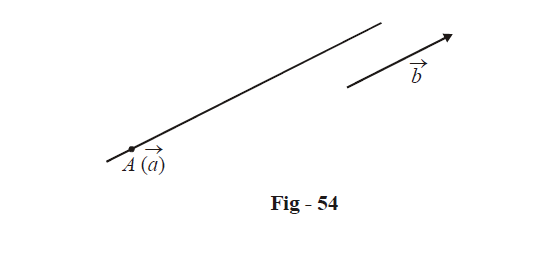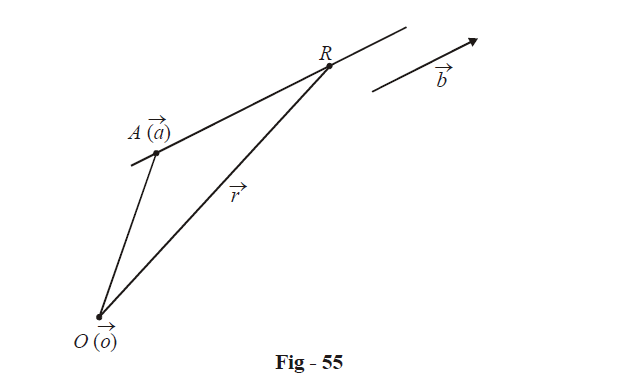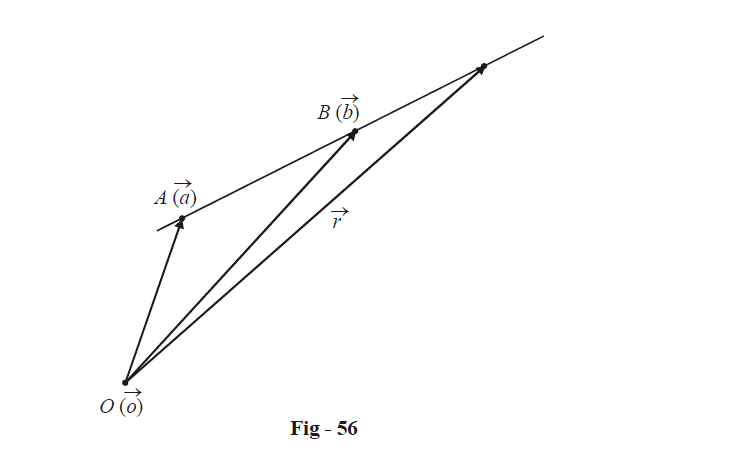# Vector Equations Of Lines

Go back to  'Vectors and 3-D Geometry'

## VECTOR EQUATIONS OF A LINE

Consider a straight line passing through the point $$A(\vec{a})$$ and parallel to the vector $$\vec{b}.$$Any point $$\vec{r}$$ on this line can be written in terms of real parameter  $$\lambda$$.\begin{align}& \vec{r}=\overrightarrow{OA}+\overrightarrow{AR} \\\\ &\;\ =\vec{a}+\lambda \vec{b}\,\,\qquad\qquad\qquad\;where\,\,\lambda \in \mathbb{R} \\ \end{align}

The equation

$\boxed{\vec r = \vec a + \lambda \vec b}$

can be viewed as the (vector) equation of this line. As we vary $$\lambda$$, we get varying position vectors $$\vec{r}$$ and hence varying points on this line.

This form of the equation of a line is called the parametricform since it involves the use of a parameter $$\lambda$$. We could also have specified the equation in non-parametric form. Observe that since $$\overrightarrow {AR}$$ is parallel to  $$\vec b,$$ we have

\begin{align}&\qquad\;\left( {\vec r - \vec a} \right) \times \vec b = \vec 0 \hfill \\&\Rightarrow \quad \boxed{\vec r \times \vec b = \vec a \times \vec b} \hfill \\ \end{align}

This is the required equation of the line. You must convince yourself that this equation is valid; in particular, understand that only points lying on the line and none other will satisfy this equation.

We can use the equations obtained above to obtained the equation of a line passing through the points $$A(\vec a)\,and\,B(\vec b).$$$\boxed{\vec r = \vec a + \lambda (\vec b - \vec a)}\qquad \qquad Parametric{\text{ }}form$

OR

\begin{align}& \qquad\;(\vec r - \vec a) \times (\vec b - \vec a) = \vec 0 \hfill \\\\&\Rightarrow \quad \boxed{\vec r \times (\vec b - \vec a) = \vec a \times \vec b}\qquad \qquad Non{\text{ }} - {\text{ }}parametric{\text{ }}form \hfill \\ \end{align}

Vectors
grade 11 | Questions Set 1
Vectors